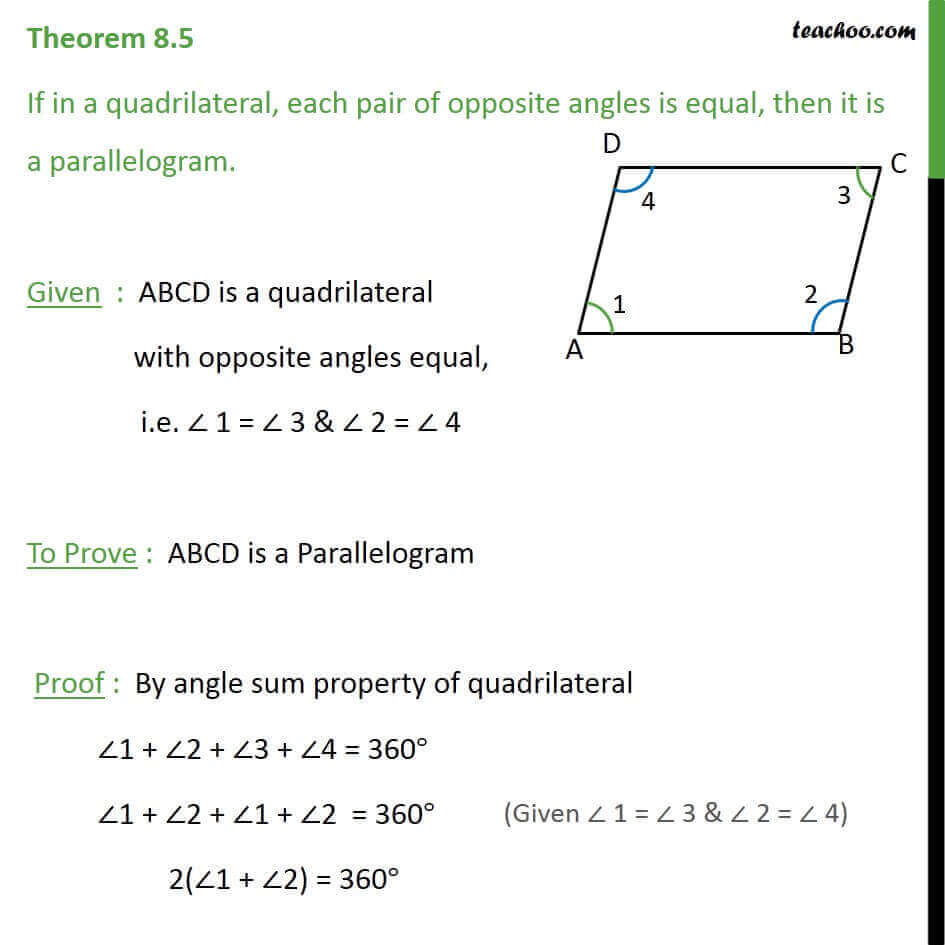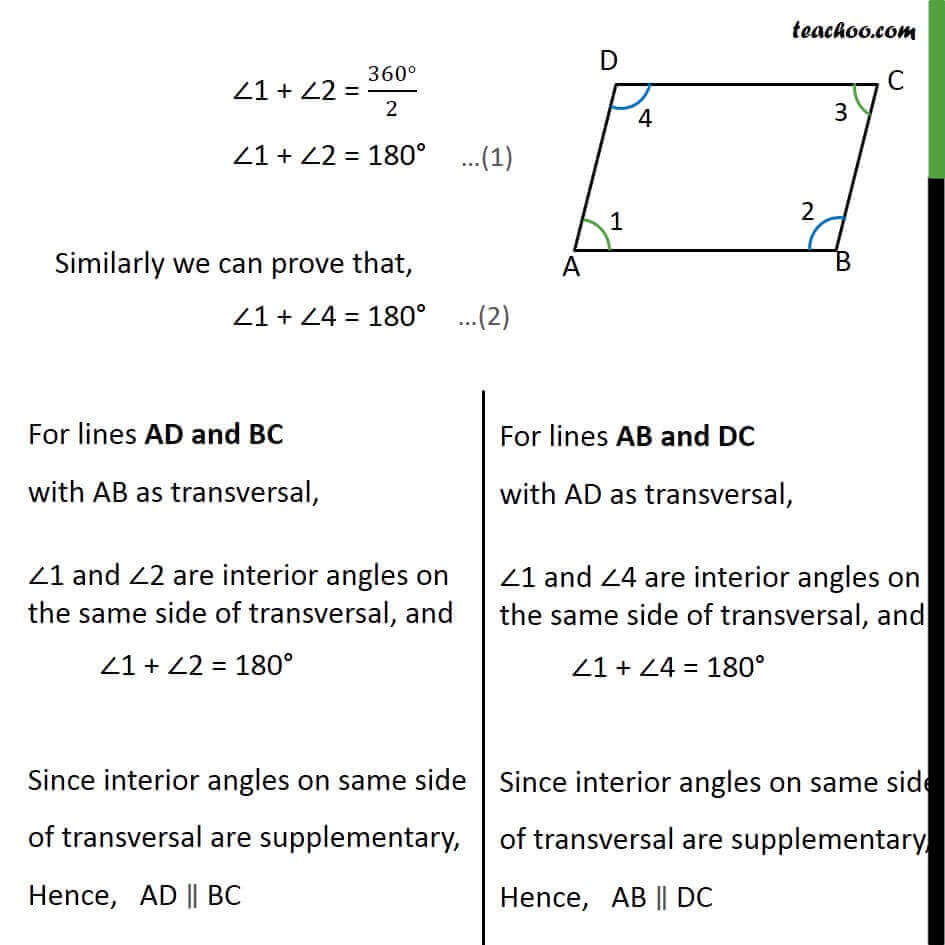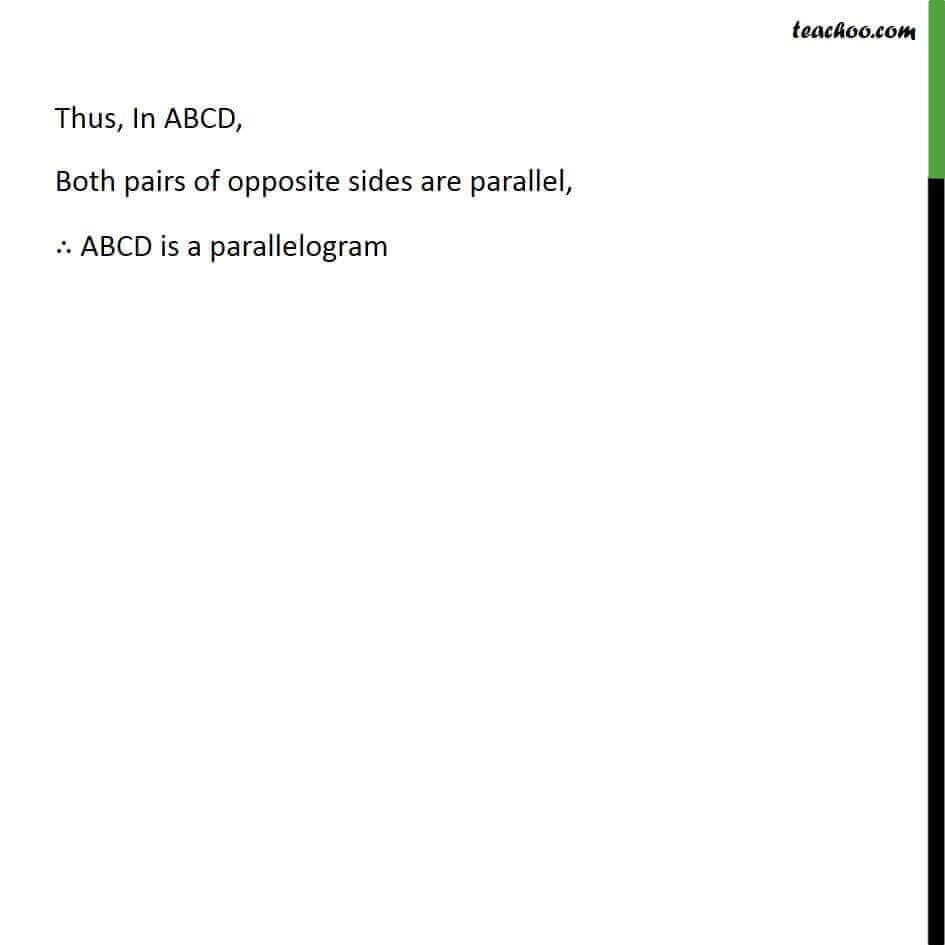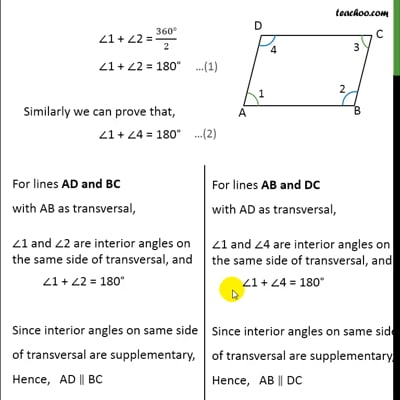Theorems

Chapter 8 Class 9 Quadrilaterals
Serial order wiseThis video is only available for Teachoo black users

Solve all your doubts with Teachoo Black (new monthly pack available now!)

### Transcript

Theorem 8.5 If in a quadrilateral, each pair of opposite angles is equal, then it is a parallelogram. Given : ABCD is a quadrilateral with opposite angles equal, i.e. 1 = 3 & 2 = 4 To Prove : ABCD is a Parallelogram Proof : By angle sum property of quadrilateral 1 + 2 + 3 + 4 = 360 1 + 2 + 1 + 2 = 360 2( 1 + 2) = 360 1 + 2 = 360 2 1 + 2 = 180 Similarly we can prove that, 1 + 4 = 180 Thus, In ABCD, Both pairs of opposite sides are parallel, ABCD is a parallelogram